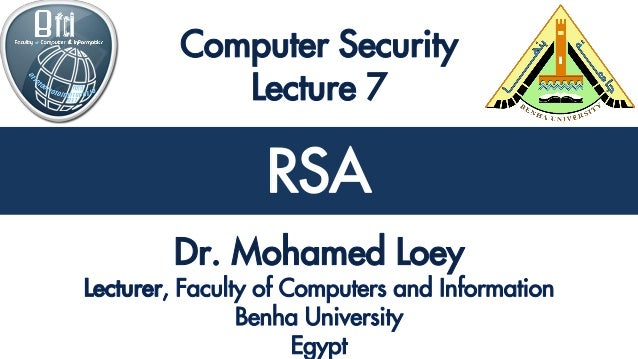Successfully reported this slideshow.Upcoming SlideShare
×

# Computer Security Lecture 7: RSA

807 views

Published on

We will discuss the following: RSA Key generation , RSA Encryption , RSA Decryption , A Real World Example, RSA Security.

Published in: Education
• Full Name
Comment goes here.

Are you sure you want to Yes No• Very nice tips on this. In case you need help on any kind of academic writing visit website ⇒ www.WritePaper.info ⇐ and place your order

Are you sure you want to  Yes  No
• Benha University http://bu.edu.eg/staff/mloey

Are you sure you want to  Yes  No

### Computer Security Lecture 7: RSA

1. 1. RSA RSA
2. 2. RSA RSA RSA Key generation RSA Encryption RSA Decryption A Real World Example RSA Security
3. 3. RSA RSA RSA Key generation RSA Encryption RSA Decryption A Real World Example RSA Security
4. 4. RSA RSA is one of the first practical public-key cryptosystems and is widely used for secure data transmission. RSA is made of the initial letters of the surnames of Ron Rivest, Adi Shamir, and Leonard Adleman, who first publicly described the algorithm in 1977.
5. 5. RSA  RSA is Asymmetric Encryption Encryption Key Decryption Key Encrypt Decrypt \$1000 \$1000%3f7&4 Two separate keys which are not shared
6. 6. RSA RSA RSA Key generation RSA Encryption RSA Decryption A Real World Example RSA Security
7. 7. RSA 1) Choose two distinct prime numbers 𝒑 and 𝒒 2) Compute 𝒏 = 𝑝 ∗ 𝑞 3) Compute φ(n) = (p - 1) * (q - 1) 4) Choose e such that 1 < e < φ(n) and e and n are prime. 5) Compute a value for d such that (d * e) % φ(n) = 1  Public key is (e, n)  Private key is (d, n)
8. 8. RSA  Choose p = 3 and q = 11  Compute n = p * q = 3 * 11 = 33  Compute φ(n) = (p - 1) * (q - 1) = 2 * 10 = 20  Choose e such that 1 < e < φ(n) and e and n are prime. Let e = 7  Compute a value for d such that (d * e) % φ(n) = 1. One solution is d = 3 [(3 * 7) % 20 = 1]  Public key is (e, n) => (7, 33)  Private key is (d, n) => (3, 33)
9. 9. RSA RSA RSA Key generation RSA Encryption RSA Decryption A Real World Example RSA Security
10. 10. RSA  m = plaintext  Public key is (e, n)  C= Ciphertext 𝐶 = 𝑚 𝑒 % 𝑛
11. 11. RSA  m = 2  Public key is (e, n) => (7, 33) C = 2 7 % 33 = 29
12. 12. RSA RSA RSA Key generation RSA Encryption RSA Decryption A Real World Example RSA Security
13. 13. RSA  C= Ciphertext  m = plaintext  Private key is (d, n) 𝑚 = 𝐶 𝑑 % 𝑛
14. 14. RSA  C= 29  Private key is (d, n) => (3, 33) 𝑚 = 𝐶3 % 33 = 2
15. 15. RSA  Select two prime numbers, p = 17 and q = 11.  Calculate n = pq = 17 * 11 = 187.  Calculate φ (n) = (p - 1)(q - 1) = 16 * 10 = 160.  Select e such that e is relatively prime to φ(n) = 160 and less than φ(n); we choose e = 7.  Determine d such that d.e = 1 (mod 160) and d < 160. The correct value is d = 23, because 23 * 7 = 161 = (1 * 160) + 1  Public Key= {7, 187} and Private Key = {23, 187}
16. 16. RSA
17. 17. RSA RSA RSA Key generation RSA Encryption RSA Decryption A Real World Example RSA Security
18. 18. RSA lets encrypt the message "attack at dawn“ Convert the message into a numeric format. Each letter is represented by an ASCII character. "attack at dawn" becomes 1976620216402300889624482718775150
19. 19. RSA  p= 12131072439211271897323671531612440428472427633701410 92563454931230196437304208561932419736532241686654101 7057361365214171711713797974299334871062829803541  q= 12027524255478748885956220793734512128733387803682075 43365389998395517985098879789986914690080913161115334 6817050832096022160146366346391812470987105415233
20. 20. RSA  n=1459067680075833232301869393490706352924018723753571643995818 7101987343879900535893836957140267014980212181808629246742282815 7022922076746906543401224889672472407926969987100581290103199317 8587536637108623576565105078837142971156373427889114635351027120 32765166518411726859837988672111837205085526346618740053  ϕ(n)=14590676800758332323018693934907063529240187237535716439958 1871019873438799005358938369571402670149802121818086292467422828 1570229220767469065434012248896483138112322799663173013977778523 6530154784827347887129722205858745715289160645926971811926897116 3555070802643999529549644116811947516513938184296683521280
21. 21. RSA  e= 65537 d=89489425009274444368228545921773093919669586065884 25744549785445648767483962981839093494197326287961679 79706089172836798754993315741611138540888132754881105 88247193077582527278437906504015680623423550067240042 46666565423238350292221549362328947213886644581878912 7946123407807725702626644091036502372545139713
22. 22. RSA  Encryption: C= 1976620216402300889624482718775150 ^e % n C= 35052111338673026690212423937053328511880760811579981 62064280234668581062310985023594304908097338624111378 40407947041939782153784997654130836464387847409523069 32534945195080183861574225226218879827232453912820596 88644037753608246568175007441745915148540744586251102 3472235560823053497791518928820272257787786
23. 23. RSA  Decryption: P= 35052111338673026690212423937053328511880760811579981620642 80234668581062310985023594304908097338624111378404079470419 39782153784997654130836464387847409523069325349451950801838 61574225226218879827232453912820596886440377536082465681750 07441745915148540744586251102347223556082305349779151892882 0272257787786 ^d % n  P= 1976620216402300889624482718775150 (which is our plaintext "attack at dawn")
24. 24. RSA RSA RSA Key generation RSA Encryption RSA Decryption A Real World Example RSA Security
25. 25. RSA  Two approaches to attacking RSA: brute force key search (infeasible given size of numbers) mathematical attacks (based on difficulty of computing ø(N), by factoring modulus N)